##### Electronics For Dummies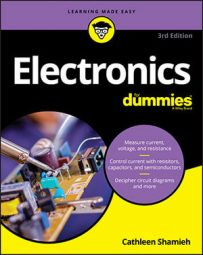With just a handful of basic mathematical formulas, you can get pretty far in analyzing the goings-on in electronic circuits and in choosing values for electronic components in circuits you design.

## Ohm’s Law and Joule’s Law

Ohm’s Law and Joule’s Law are commonly used in calculations dealing with electronic circuits. These laws are straightforward, but when you’re trying to solve for one variable or another, it is easy to get them confused. The following table presents some common calculations using Ohm’s Law and Joule’s Law. In these calculations:

V = voltage (in volts)

I = current (in amps)

R = resistance (in ohms)

P = power (in watts)

Unknown Value Formula
Voltage V = I x R
Current I = V/R
Resistance R = V/I
Power P = V x I or P = V2/R or P = I2R

## Equivalent resistance and capacitance formulas

Electronic circuits may contain resistors or capacitors in series, parallel, or a combination. You can determine the equivalent value of resistance or capacitance using the following formulas:

Resistors in series: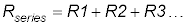Resistors in parallel: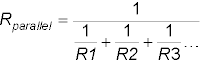or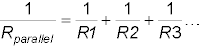Capacitors in series: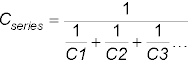or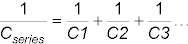Capacitors in parallel: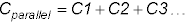## Kirchhoff’s Current and Voltage Laws

Kirchhoff’s Circuit Laws are commonly used to analyze what’s going on in a closed loop circuit. Based on the principle of conservation of energy, Kirchhoff’s Current Law (KCL) states that, at any node (junction) in an electrical circuit, the sum of currents flowing into that node is equal to the sum of currents flowing out of that node, and Kirchhoff’s Voltage Law (KVL) states that the sum of all voltage drops around a circuit loop equals zero.

For the circuit shown, Kirchhoff’s Laws tells you the following:

KCL: I = I1 + I2

KVL: Vbattery - VR - VLED = 0, or Vbattery = VR + VLED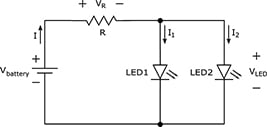## Calculating the RC time constant

In a resistor-capacitor (RC) circuit, it takes a certain amount of time for the capacitor to charge up to the supply voltage, and then, once fully charged, to discharge down to 0 volts.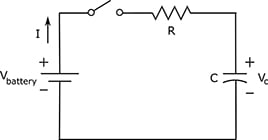Circuit designers use RC networks to produce simple timers and oscillators because the charge time is predictable and depends on the values of the resistor and the capacitor. If you multiply R (in ohms) by C (in farads), you get what is known as the RC time constant of your RC circuit, symbolized by T: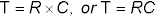A capacitor charges and discharges almost completely after five times its RC time constant, or 5RC. After the equivalent of one time constant has passed, a discharged capacitor will charge to roughly two-thirds its capacity, and a charged capacitor will discharge nearly two-thirds of the way.